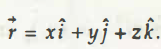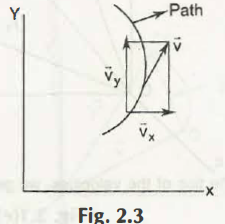Suppose there is a graph based on the x, y, z coordinates. From that graph, there is an equation, which is,

Referring from the same graph again, we get another equation,On differentiating the above equation (2.2),

(Equation 2.3)

From the equation (2.3) you will get the scalar component of velocity which is,

vx = x , vy = y and vz = z(Equation 2.4)

From the above equation, scalar components of accelerations,

ax= , ay = , az=

, ,

, ,Links of Next Mechanical Engineering Topics:-### Customer Reviews

My Homework Help
Rated 5.0 out of 5 based on 510 customer reviews at